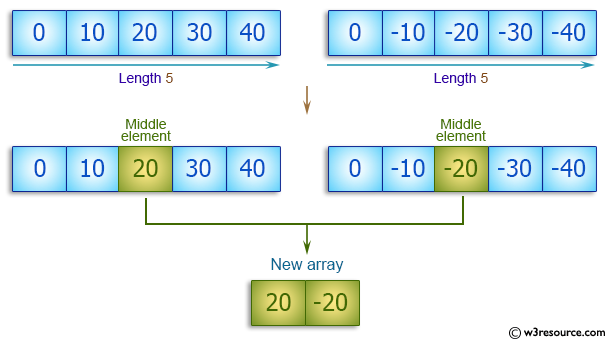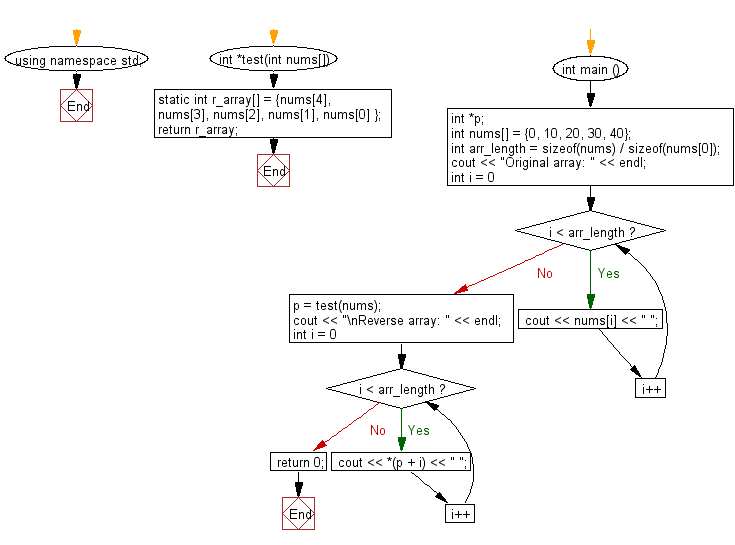﻿ C++ : New array using the middle elements from two arrays# C++ Exercises: Create a new array containing the middle elements from the two given arrays of integers, each length 5

## C++ Basic Algorithm: Exercise-87 with Solution

Write a C++ program to create a new array containing the middle elements from the two given arrays of integers, each length 5.

Sample Solution:

C++ Code :

``````#include <iostream>
using namespace std;

int *test(int nums1[], int nums2[]) {

static int  max_array[] = { nums1, nums2 };
return max_array;
}

int main () {
// a pointer to an int.
int *p;
int nums1[] = {0, 10, 20, 30, 40};
int nums2[] = {0, -10, -20, -30, -40};

p = test(nums1, nums2);

cout << "\nNew array: " << endl;
for ( int i = 0; i < 2; i++ ) {
cout << *(p + i) << " ";
}

return 0;
}
``````

Sample Output:

```New array:
20 -20
```

Pictorial Presentation:Flowchart:C++ Code Editor:

Contribute your code and comments through Disqus.

What is the difficulty level of this exercise?

﻿